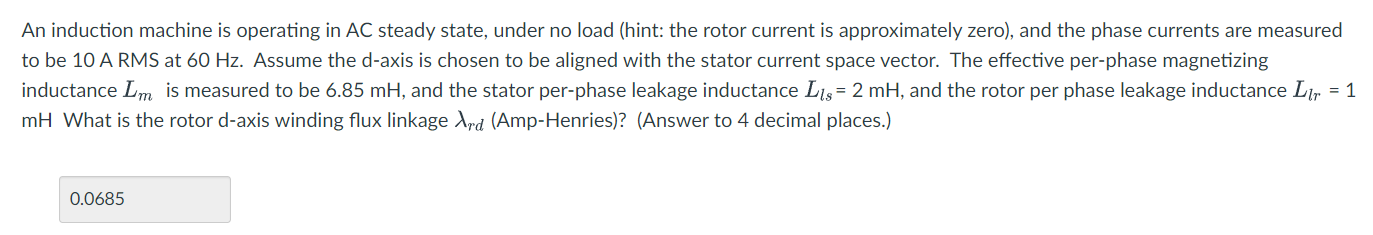# (Solved): An induction machine is operating in AC steady state, under no load (hint: th ...​​​​​​​

An induction machine is operating in AC steady state, under no load (hint: the rotor current is approximately zero), and the phase currents are measured to be at . Assume the d-axis is chosen to be aligned with the stator current space vector. The effective per-phase magnetizing inductance is measured to be , and the stator per-phase leakage inductance , and the rotor per phase leakage inductance What is the rotor d-axis winding flux linkage (Amp-Henries)? (Answer to 4 decimal places.)

We have an Answer from Expert

==> Given Information We are given the following information:
The induction machine is operating in AC steady state.
The machine is under no-load conditions, which means the rotor current is approximately zero.
The phase currents measured on the stator side are 10 A RMS at 60 Hz.
The effective per-phase magnetizing inductance is Lm = 6.85 mH.
The stator per-phase leakage inductance is Ls = 2 mH.
The rotor per-phase leakage inductance is Lr = 1 mH.
Calculating Peak Stator Phase Current :

To determine the peak value of the stator phase current, we multiply the RMS value by the square root of 2.

In this case, the peak value is calculated as follows:

We have an Answer from Expert